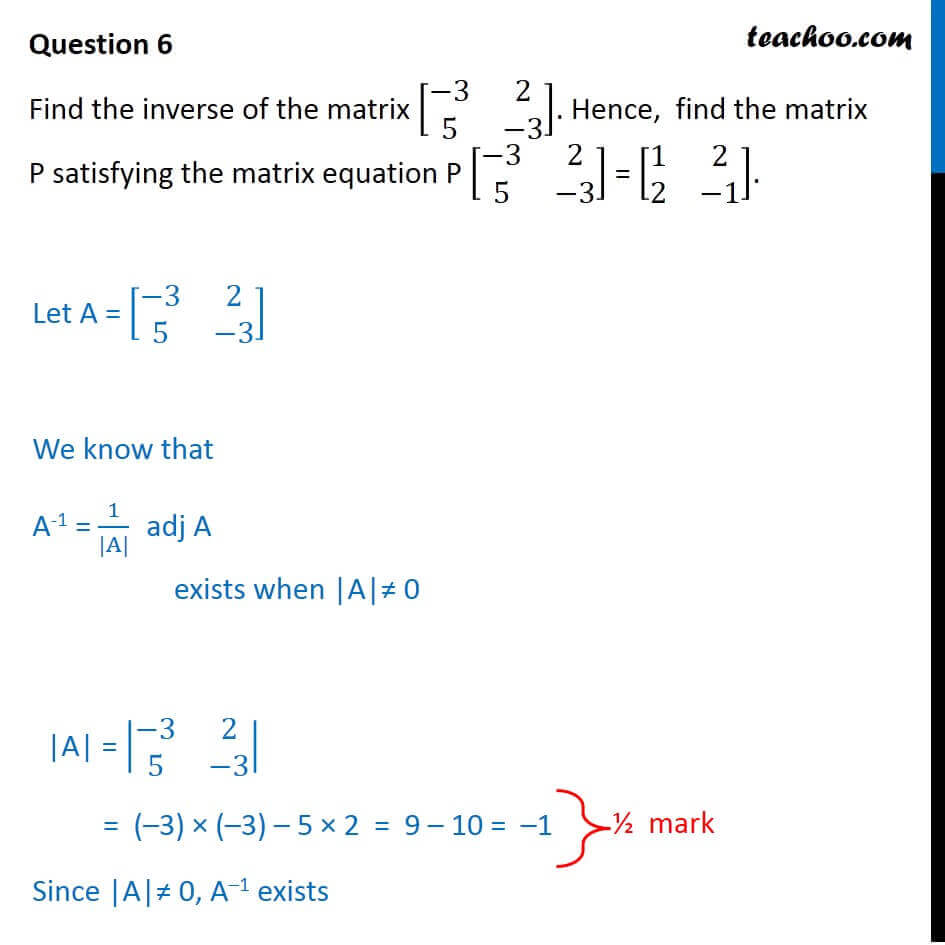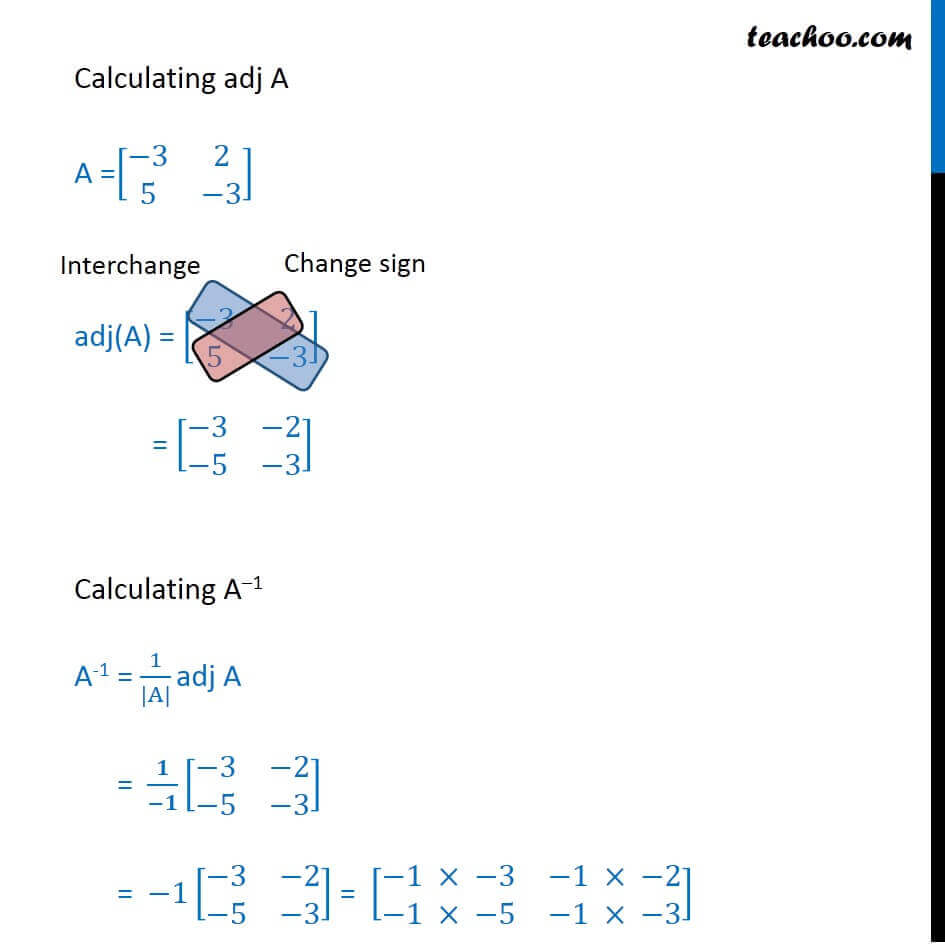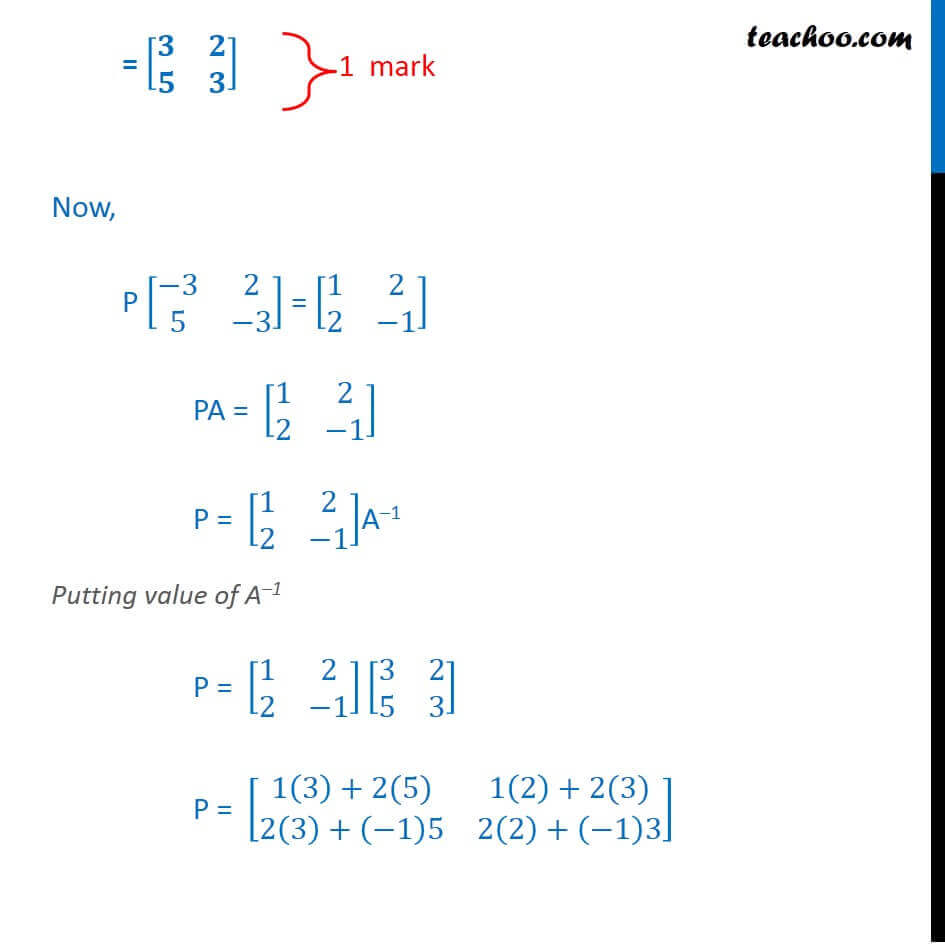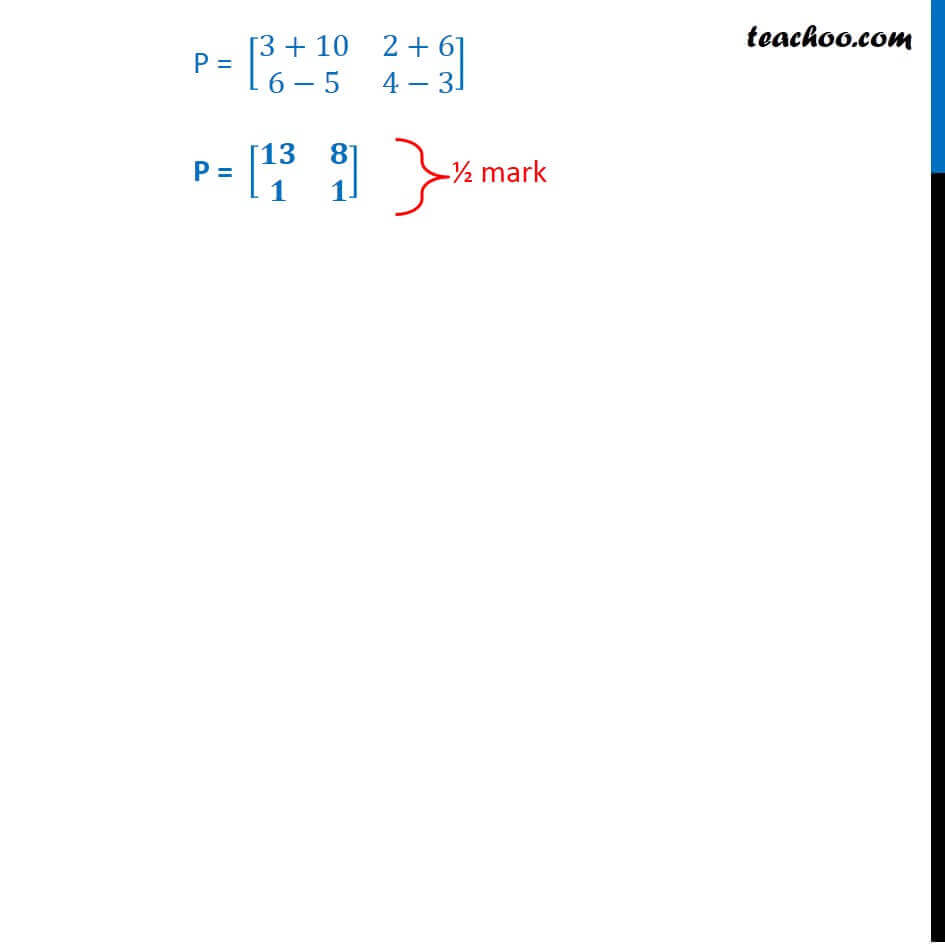CBSE Class 12 Sample Paper for 2018 Boards

Class 12
Solutions of Sample Papers and Past Year Papers - for Class 12 Boards

### Find the inverse of the matrix [-3 2 5 -3] . Hence,  find the matrix P satisfying the matrix equation P [-3 2 5 -3] = [1 2 2 -1] .

This is a question of CBSE Sample Paper - Class 12 - 2017/18.Learn in your speed, with individual attention - Teachoo Maths 1-on-1 Class

### Transcript

Question 6 Find the inverse of the matrix [■8(−3&2@5&−3)]. Hence, find the matrix P satisfying the matrix equation P [■8(−3&2@5&−3)] = [■8(1&2@2&−1)]. Let A = [■8(−3&2@5&−3)] We know that A-1 = 1/(|A|) adj A exists when |A|≠ 0 |A| = |■8(−3&2@5&−3)| = (–3) × (–3) – 5 × 2 = 9 – 10 = –1 Since |A|≠ 0, A–1 exists Calculating adj A A =[■8(−3&2@5&−3)] adj(A) = [■8(−3&2@5&−3)] = [■8(−3&−2@−5&−3)] Calculating A–1 A-1 = 1/(|A|) adj A = 𝟏/(−𝟏) [■8(−3&−2@−5&−3)] = −1[■8(−3&−2@−5&−3)] = [■8(−1 × −3&−1 × −2@−1 × −5&−1 × −3)] = [■8(𝟑&𝟐@𝟓&𝟑)] Now, P [■8(−3&2@5&−3)] = [■8(1&2@2&−1)] PA = [■8(1&2@2&−1)] P = [■8(1&2@2&−1)]A–1 Putting value of A–1 P = [■8(1&2@2&−1)][■8(3&2@5&3)] P = [■8(1(3)+2(5)&1(2)+2(3)@2(3)+(−1)5&2(2)+(−1)3)] P = [■8(3+10&2+6@6−5&4−3)] P = [■8(𝟏𝟑&𝟖@𝟏&𝟏)]